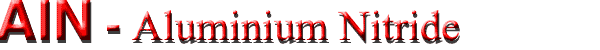## Thermal properties

### Basic parameters

 Wurtzite crystal structure Remarks Referens Bulk modulus 21 x 1011 dyn cm -2 Goldberg (2001) Debye temperature 1150 K Melting point 3273 K MacChesney et al. (1970) 3023 K (between 100 and 500 atm of nitrogen) Goldberg (2001) Specific heat 0.6 J g-1°C -1 Thermal diffusivity 1.47 cm2 s-1 Thermal conductivity 2.85 W cm-1 °C -1 300 K. experiment Slack et al. (1987) Thermal expansion coefficient, linear αort = αc = 5.27 x10-6 K-1 T=20...800 °C. X-ray, epitaxial layers Sirota & Golodushko (1974) also see Qian et al. (1996) α|| = αa = 4.15 x10-6 K-1

### Thermal conductivity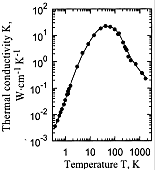AlN, Wurtzite sructure. The thermal conductivity K vs. temperature for high-purity AlN. Experimental data. Slack et al. (1987)AlN, Wurtzite sructure. The thermal conductivity K vs. temperature. 1 -- AlN single crystal with no oxygen and a sample diameter of 0.54 cm (estimate); 2 -- AlN single crystal containing N0 ~= 4.2 x 1019 cm-3 oxygen atoms (measure); 3 -- N0 ~= 3 x 1020 cm-3 oxygen atoms. Slack et al. (1987)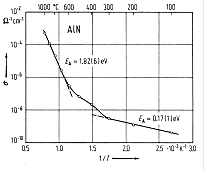AlN, Wurtzite sructure. Conductivity vs. reciprocal temperature for hot-pressed material. EA: activation energy for conductivity. Francis & Worrell (1976)
 At 0.4 < T < 3 K: K ~ T2.54 At 500 < T < 1800 K: K ~ T-1.25
Maximal measured value of thermal conductivity at 300 K is 2.85 W cm-1 K-1 [Slack et al. (1987)].

The specific heat Cp of AlN at constant pressure
 At 300< T< 1800K, Cp = 45.94 + 3.347 x 10-3 x T -14.98 x 105 x T -2 (J mol-1 K) Koshchenko et al. (1984) At 1800 < T < 2700 K, Cp = 37.34 + 7.866 x 10-3 x T (J mol-1 K)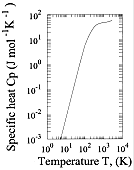AlN. The specific heat vs. temperature at constant pressure. Koshchenko et al. (1984)

### Thermal Expansion

For the direction along the c-axis:
 At 300 :  αc = 5.3 x 10-6 K-1 Slack and Bartram (1975), Touloukian at al. (1977), Meng (1994), Morkoc et al. (1994)  Qian et al. (1996) At 293 < T < 1700 K,   Δc/c300 = -7.006 x 10-2 + 1.583 x 10-4 x T + 2.719 x 10-7 x T2 - 5.834 x 10-11 x T3

For the perpendiculardiraection:
 At 300 :    αa = 4.2 x 10-6 K-1 Slack and Bartram (1975), Touloukian at al. (1977), Meng (1994), Morkoc et al. (1994)  Qian et al. (1996) At 293 < T < 1700 K,   Δa/a300 = -8.679 x 10-2 + 1.929 x 10-4 x T + 3.400 x 10-7 x T2 -7.969 x 10-11 x T3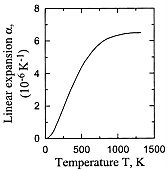AlN. The linear expansion coefficient α vs. temperature for ceramic AlN samples. α = 1/3 (Δc/c + 2Δa/a) Slack & Bartram (1975)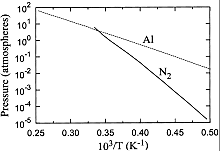Calculated vapor pressure of Al and N2 in equilibrium with solid AlN and liquid Al. Meng (1994)